# Minimum Coins (DP – 20)

Problem Statement:  Minimum Coins

Problem Link: Minimum Coins

We are given a target sum of ‘X’ and ‘N’ distinct numbers denoting the coin denominations. We need to tell the minimum number of coins required to reach the target sum. We can pick a coin denomination for any number of times we want.

```Example: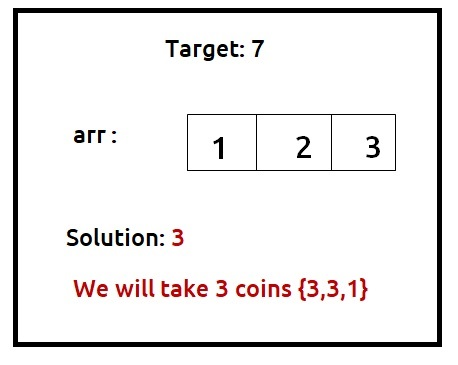```

Disclaimer: Don’t jump directly to the solution, try it out yourself first.

Pre-req: Subset Sum equal to the target

### Solution :

Why a Greedy Solution doesn’t work?

The first approach that comes to our mind is greedy. A greedy solution will fail in this problem because there is no ‘uniformity’ in data. While selecting a local better choice we may choose an item that will in the long term give less value.

Let us understand this with help of an example: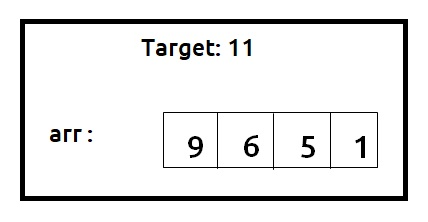A Greedy solution will be to take the highest denomination coin first, so we will take an item on index 0, with a value of 9. Now the remaining target sum will be 2. Therefore we can only consider coins with a value of 1. We will take 2 coins of value 1 to meet the target. So a greedy solution gives us the answer 3 {9,1,1}.

Now we can clearly see that a non-greedy solution of taking 2 coins valued 6 and 5 will give us a better option. So we can say that the greedy solution doesn’t work for this problem.

As the greedy approach doesn’t work, we will try to generate all possible combinations using recursion and select the combination which gives us the minimum number of coins.

Steps to form the recursive solution:

We will first form the recursive solution by the three points mentioned in Dynamic Programming Introduction

Step 1: Express the problem in terms of indexes.

We are given ‘n’ distinct numbers. Their denomination is represented by the ‘arr’ array. So clearly one parameter will be ‘ind’, i.e index up to which the array items are being considered.

There is one more parameter “T”. We need to know the given target that we want to achieve.

So, we can say that initially, we need to find f(n-1, T) where T is the initial target given to us. f(n-1, T) means we are finding the minimum number of coins required to form the target sum by considering coins from index 0 to n-1.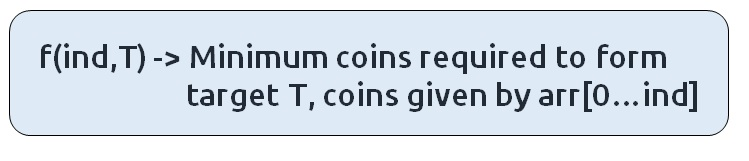Base Cases:

If ind==0, it means we are at the first item, so in that case, the following cases can arise:

• arr = 4 and T = 12

In such a case where the target is divisible by the coin element, we will return T%arr.

• arr =4 and T=1 , arr=3 T=10

In all other cases, we will not be able to form a solution, so we will return a big number like 1e9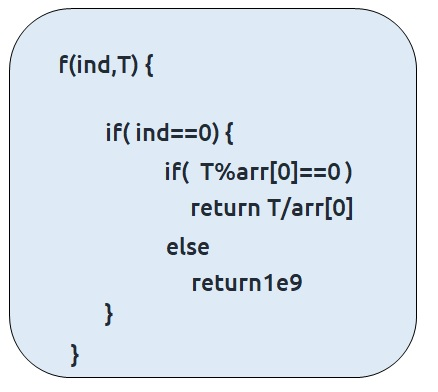Step 2: Try out all possible choices at a given index.

We need to generate all the subsequences. We will use the pick/non-pick technique as discussed in this video “Recursion on Subsequences”. There will be a slight change for this question which is discussed below.

We have two choices:

• Exclude the current element in the subsequence: We first try to find a subsequence without considering the current index coin. If we exclude the current coin, the target sum will not be affected and the number of coins added to the solution will be 0. So we will call the recursive function f(ind-1,T)
• Include the current element in the subsequence: We will try to find a subsequence by considering the current icoin. As we have included the coin, the target sum will be updated to T-arr[ind] and we have considered 1 coin to our solution.

Now here is the catch, as there is an unlimited supply of coins, we want to again form a solution with the same coin value. So we will not recursively call for f(ind-1, T-arr[ind]) rather we will stay at that index only and call for f(ind, T-arr[ind]) to find the answer.
Note: We will consider the current coin only when its denomination value (arr[ind]) is less than or equal to the target T.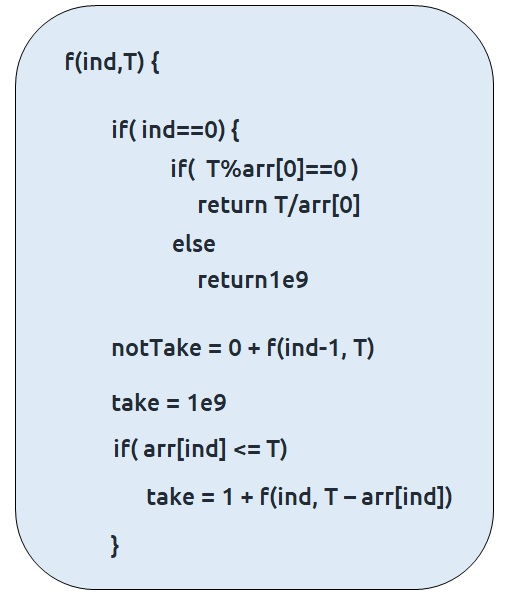Step 3:  Return the minimum of take and notTake

As we have to return the minimum number of coins, we will return the minimum of take and notTake as our answer.

The final pseudocode after steps 1, 2, and 3: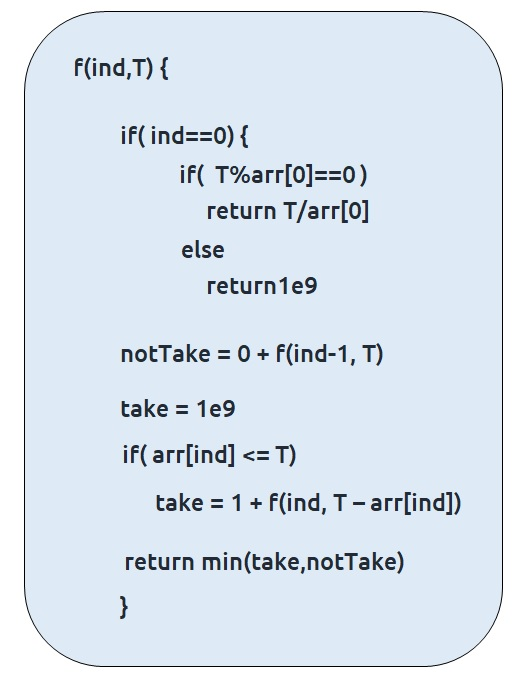Steps to memoize a recursive solution:

If we draw the recursion tree, we will see that there are overlapping subproblems. In order to convert a recursive solution the following steps will be taken:

1. Create a dp array of size [n][T+1]. The size of the input array is ‘N’, so the index will always lie between ‘0’ and ‘n-1’. The target Sum can take any value between ‘0’ and ‘T’. Therefore we take the dp array as dp[n][T+1]
2. We initialize the dp array to -1.
3. Whenever we want to find the answer of particular parameters (say f(ind,T)), we first check whether the answer is already calculated using the dp array(i.e dp[ind][T]!= -1 ). If yes, simply return the value from the dp array.
4. If not, then we are finding the answer for the given value for the first time, we will use the recursive relation as usual but before returning from the function, we will set dp[ind][target] to the solution we get.

Code:

## C++Code

``````#include <bits/stdc++.h>

using namespace std;

int minimumElementsUtil(vector<int>& arr, int ind, int T, vector<vector<int>>& dp){

if(ind == 0){
if(T%arr == 0) return T/arr;
else return 1e9;
}

if(dp[ind][T]!=-1)
return dp[ind][T];

int notTaken = 0 + minimumElementsUtil(arr,ind-1,T,dp);

int taken = 1e9;
if(arr[ind] <= T)
taken = 1 + minimumElementsUtil(arr,ind,T-arr[ind],dp);

return dp[ind][T] = min(notTaken,taken);
}

int minimumElements(vector<int>& arr, int T){

int n= arr.size();

vector<vector<int>> dp(n,vector<int>(T+1,-1));
int ans =  minimumElementsUtil(arr, n-1, T, dp);
if(ans >= 1e9) return -1;
return ans;
}

int main() {

vector<int> arr ={1,2,3};
int T=7;

cout<<"The minimum number of coins required to form the target sum is "
<<minimumElements(arr,T);
}
``````

Output:

The minimum number of coins required to form the target sum is 3

Time Complexity: O(N*T)

Reason: There are N*T states therefore at max ‘N*T’ new problems will be solved.

Space Complexity: O(N*T) + O(N)

Reason: We are using a recursion stack space(O(N)) and a 2D array ( O(N*T)).

## Java Code

``````import java.util.*;

class TUF{
static int minimumElementsUtil(int[] arr, int ind, int T, int[][] dp){

if(ind == 0){
if(T%arr == 0) return T/arr;
else return (int)Math.pow(10,9);
}

if(dp[ind][T]!=-1)
return dp[ind][T];

int notTaken = 0 + minimumElementsUtil(arr,ind-1,T,dp);

int taken = (int)Math.pow(10,9);
if(arr[ind] <= T)
taken = 1 + minimumElementsUtil(arr,ind,T-arr[ind],dp);

return dp[ind][T] = Math.min(notTaken,taken);
}

static int minimumElements(int[] arr, int T){

int n= arr.length;

int[][] dp=new int[n][T+1];

for(int row[]: dp)
Arrays.fill(row,-1);

int ans =  minimumElementsUtil(arr, n-1, T, dp);
if(ans >= (int)Math.pow(10,9)) return -1;
return ans;
}

public static void main(String args[]) {

int arr[] ={1,2,3};
int T=7;

System.out.println("The minimum number of coins required to form the target sum is
"+minimumElements(arr,T));
}
}``````

Output:

The minimum number of coins required to form the target sum is 3

Time Complexity: O(N*T)

Reason: There are N*T states therefore at max ‘N*T’ new problems will be solved.

Space Complexity: O(N*T) + O(N)

Reason: We are using a recursion stack space(O(N)) and a 2D array ( O(N*T)).

Steps to convert Recursive Solution to Tabulation one.

To convert the memoization approach to a tabulation one, create a dp array with the same size as done in memoization. We can initialize it as 0.

First, we need to initialize the base conditions of the recursive solution.

• At ind==0, we are considering the first element, if T%arr ==0, we initialize it to T/arr else we initialize it to 1e9.
• Next, we are done for the first row, so our ‘ind’ variable will move from 1 to n-1, whereas our ‘target’ variable will move from 0 to ‘T’. We will set the nested loops to traverse the dp array.
• Inside the nested loops we will apply the recursive logic to find the answer of each cell.
• When the nested loop execution has ended, we will return dp[n-1][T] as our answer.

Code:

## C++ Code

``````#include <bits/stdc++.h>

using namespace std;

int minimumElements(vector<int>& arr, int T){

int n= arr.size();

vector<vector<int>> dp(n,vector<int>(T+1,0));

for(int i=0; i<=T; i++){
if(i%arr == 0)
dp[i] = i/arr;
else dp[i] = 1e9;
}

for(int ind = 1; ind<n; ind++){
for(int target = 0; target<=T; target++){

int notTake = 0 + dp[ind-1][target];
int take = 1e9;
if(arr[ind]<=target)
take = 1 + dp[ind][target - arr[ind]];

dp[ind][target] = min(notTake, take);
}
}

int ans = dp[n-1][T];
if(ans >=1e9) return -1;
return ans;
}

int main() {

vector<int> arr ={1,2,3};
int T=7;

cout<<"The minimum number of coins required to form the target sum is "
<<minimumElements(arr,T);
}
``````

Output:

The minimum number of coins required to form the target sum is 3

Time Complexity: O(N*T)

Reason: There are two nested loops

Space Complexity: O(N*T)

Reason: We are using an external array of size ‘N*T’. Stack Space is eliminated.

## Java Code

``````import java.util.*;

class TUF{
static int minimumElements(int[] arr, int T){

int n= arr.length;

int dp[][]=new int[n][T+1];

for(int i=0; i<=T; i++){
if(i%arr == 0)
dp[i] = i/arr;
else dp[i] = (int)Math.pow(10,9);
}

for(int ind = 1; ind<n; ind++){
for(int target = 0; target<=T; target++){

int notTake = 0 + dp[ind-1][target];
int take = (int)Math.pow(10,9);
if(arr[ind]<=target)
take = 1 + dp[ind][target - arr[ind]];

dp[ind][target] = Math.min(notTake, take);
}
}

int ans = dp[n-1][T];
if(ans >=(int)Math.pow(10,9)) return -1;
return ans;
}

public static void main(String args[]) {

int arr[] ={1,2,3};
int T=7;

System.out.println("The minimum number of coins required to form the target sum is
"+minimumElements(arr,T));
}
}``````

Output:

The minimum number of coins required to form the target sum is 3

Time Complexity: O(N*T)

Reason: There are two nested loops

Space Complexity: O(N*T)

Reason: We are using an external array of size ‘N*T’. Stack Space is eliminated.

Part 3: Space Optimization

If we closely look the relation,

dp[ind][target] =  dp[ind-1][target] + dp[ind-1][target-arr[ind]]

We see that to calculate a value of a cell of the dp array, we need only the previous row values (say prev). So, we don’t need to store an entire array. Hence we can space optimize it.

Note: We first need to initialize the first row as we had done in the tabulation approach.

Code:

## C++ Code

``````#include <bits/stdc++.h>

using namespace std;

int minimumElements(vector<int>& arr, int T){

int n= arr.size();

vector<int> prev(T+1,0), cur(T+1,0);

for(int i=0; i<=T; i++){
if(i%arr == 0)
prev[i] = i/arr;
else prev[i] = 1e9;
}

for(int ind = 1; ind<n; ind++){
for(int target = 0; target<=T; target++){

int notTake = 0 + prev[target];
int take = 1e9;
if(arr[ind]<=target)
take = 1 + cur[target - arr[ind]];

cur[target] = min(notTake, take);
}
prev = cur;
}

int ans = prev[T];
if(ans >=1e9) return -1;
return ans;
}

int main() {

vector<int> arr ={1,2,3};
int T=7;

cout<<"The minimum number of coins required to form the target sum is "
<<minimumElements(arr,T);
}
``````

Output:

The minimum number of coins required to form the target sum is 3

Time Complexity: O(N*T)

Reason: There are two nested loops.

Space Complexity: O(T)

Reason: We are using two external arrays of size ‘T+1’.

## Java Code

``````import java.util.*;

class TUF{
static int minimumElements(int[] arr, int T){

int n= arr.length;

int prev[]=new int[T+1];
int cur[] =new int[T+1];

for(int i=0; i<=T; i++){
if(i%arr == 0)
prev[i] = i/arr;
else prev[i] = (int)Math.pow(10,9);
}

for(int ind = 1; ind<n; ind++){
for(int target = 0; target<=T; target++){

int notTake = 0 + prev[target];
int take = (int)Math.pow(10,9);
if(arr[ind]<=target)
take = 1 + cur[target - arr[ind]];

cur[target] = Math.min(notTake, take);
}
prev = cur;
}

int ans = prev[T];
if(ans >=(int)Math.pow(10,9)) return -1;
return ans;
}

public static void main(String args[]) {

int arr[] ={1,2,3};
int T=7;

System.out.println("The minimum number of coins required to form the target sum is
"+minimumElements(arr,T));
}
}``````

Output:

The minimum number of coins required to form the target sum is 3

Time Complexity: O(N*T)

Reason: There are two nested loops.

Space Complexity: O(T)

Reason: We are using two external arrays of size ‘T+1’.

Special thanks to Anshuman Sharma for contributing to this article on takeUforward. If you also wish to share your knowledge with the takeUforward fam, please check out this article